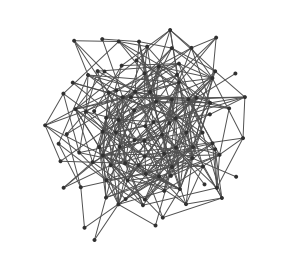# Random graph generator

Generate a random graph of any size.## Usage

This generator creates random graphs of any size. Calling `begin()` put one unique node in the graph, then `nextEvents()` will add a new node each time it is called and connect this node randomly to others.

The generator tries to generate nodes with random connections, with each node having in average a given degree. The law in a Poisson law, however, the way this generator works, adding node after node, perturbs this process. We should first allocate all the needed nodes, then create edges. However, we create nodes at the same rate as edges. The more nodes are added the more the degree distribution curve is shifted toward the right.

This generator has the ability to add randomly chosen numerical values on arbitrary attributes on edges or nodes of the graph, and to randomly choose a direction for edges.

A list of attributes can be given for nodes and edges. In this case each new node or edge added will have this attribute and the value will be a randomly chosen number. The range in which these numbers are chosen can be specified.

By default, edges are not oriented. It is possible to ask orientation, in which case the direction is chosen randomly.

## Complexity

At each call to `nextEvents()` at max k operations are run with k the average degree.

## Example

Here is a simple example generating 100 nodes with an average degree of 2: